# Division Worksheets Grade 3

Download Division Worksheets Grade 3Q.1) Divide 56 by 2 using long division method.
a) 24
b) 26
c) 28

Q.2) Divide 324 by 2 using long division method.
a) 180
b) 172
c) 162

Q.3) Divide 480 by 20 using long division method ?
a) 26
b) 34
c) 24

Q.4) 315 plants to be planted equally in a field in 5 rows. Find the number of plants to be planted in each row ?
a) 65
b) 63
c) 67

### Division Worksheets Grade 3 Explanations

Q.1) Explanation –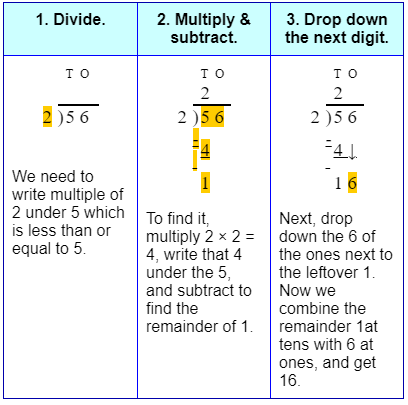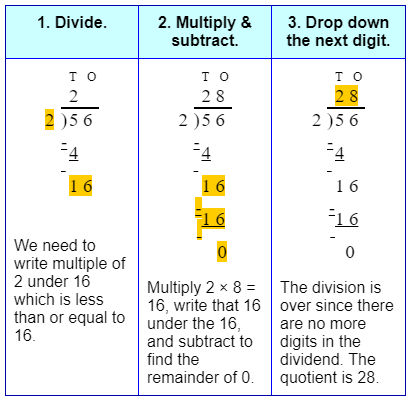Correct Answer – c) 28

Q.2) Explanation –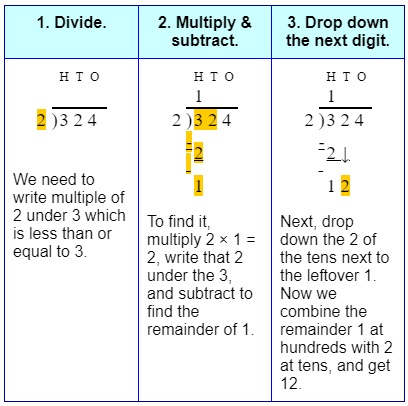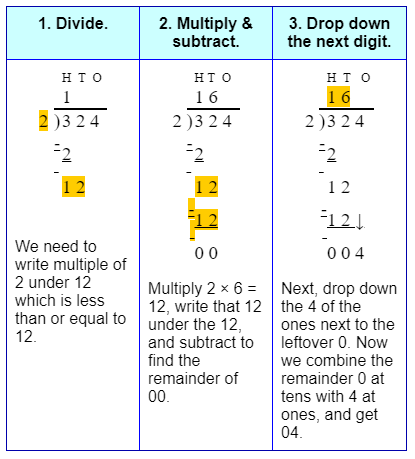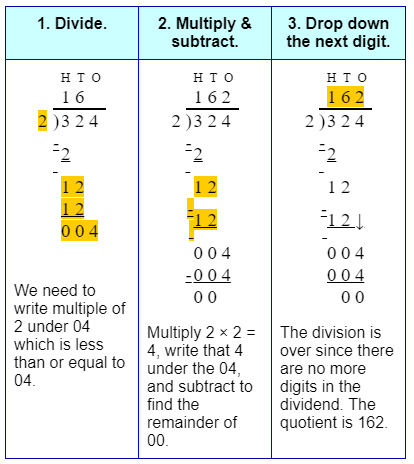Correct Answer – c) 162

Q.3) Explanation –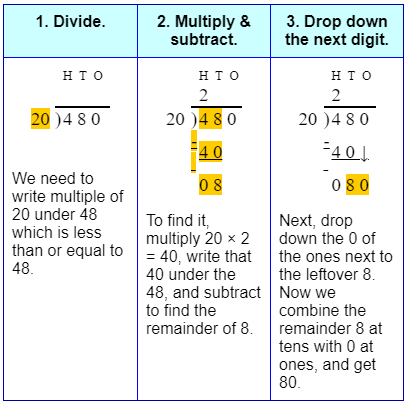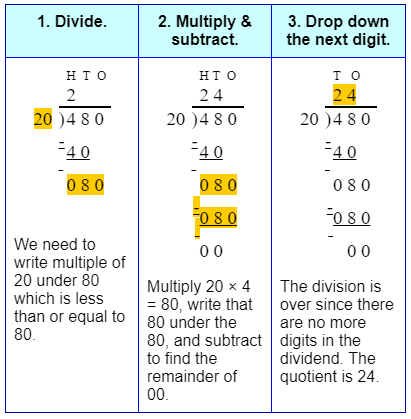Correct Answer – c) 24

Q.4) Explanation –

Number of plants to be planted = 315
Total number of rows = 5
To find the number of plants in each row we need to divide Total number of plants by Total number of rows 315 ÷ 5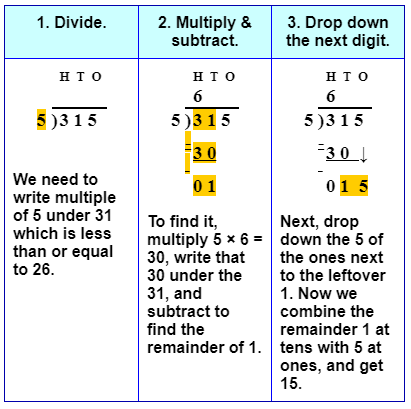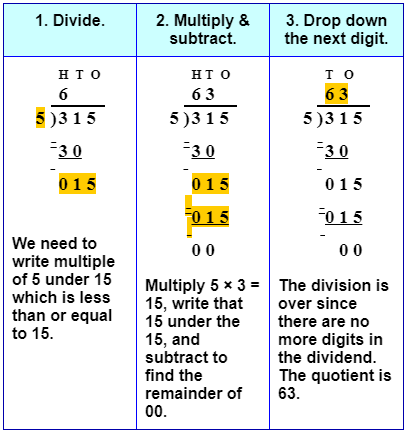So, the number of plants to be planted in each row is 63.

Correct Answer – b) 63